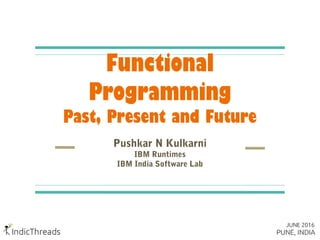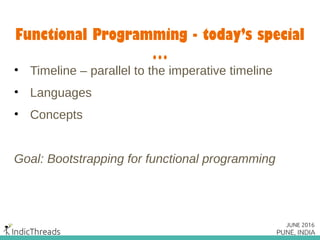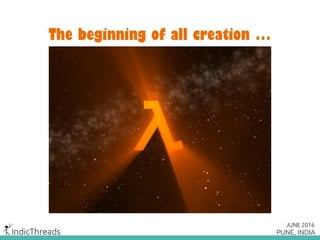Successfully reported this slideshow.

# Functional Programming Past Present Future×

# Functional Programming Past Present Future

Presented at the IndicThreads.com Software Development Conference 2016 held in Pune, India. More at http://www.IndicThreads.com and http://Pune16.IndicThreads.com

--

Presented at the IndicThreads.com Software Development Conference 2016 held in Pune, India. More at http://www.IndicThreads.com and http://Pune16.IndicThreads.com

--

### Functional Programming Past Present Future

1. 1. Functional Programming Past, Present and Future Pushkar N Kulkarni IBM Runtimes IBM India Software Lab
2. 2. Functional Programming - today’s special … • Timeline – parallel to the imperative timeline • Languages • Concepts Goal: Bootstrapping for functional programming
3. 3. The beginning of all creation …
4. 4. Theory of Computation <- Math + Logic Alonzo Church Lambda Calculus Alan Turing Turing Machine Stephen Kleene Recursive Function Theory
5. 5. Lambda Calculus It’s a formal system in mathematical logic for expressing computation based on function abstraction and application using variable binding and substitution. ~ Wikipedia
6. 6. Lambda Calculus – Fundamental Idea x //variable t //lambda term Λx.t //lambda abstraction Λx. x2 +1 // x is bound in t by Λx Λx. x2 +1(2) = 22 +1 = 5 //application Note: A lambda abstraction has no name
7. 7. LISP - 1958 LIST Processor Influenced by Church’s lambda calculus notation Multi-paradigm, pre-fix notation John McCarthy
8. 8. LISP ;;Employee compensation (defun total (bonus-calc base) (+ (funcall bonus-calc base) base)) ;; Good month (total #'(lambda (x)(* 0.8 x)) 10000) ;; Bad month (total #'(lambda (x)(+ (* 0.2 x) 10)) 10000)
9. 9. LISP – Well known dialects • Scheme – 1970 • Common Lisp – 1984 • Racket – 1984 • Clojure – 2007 • Lisp Flavoured Erlang (LFE) – 2008 (v1 March 2016)
10. 10. Academic Research (1960 – 1985) • ISWIM – If You See What I Mean – 1966 • SASL – St. Andrews Static Language – 1972 • ML – MetaLanguage – 1973 => Ocaml, F# • Miranda – 1985 => Haskell
11. 11. Back to some Lambda Calculus F(x) = x3 +4x //F(x) id a first-order function //maps values to values dF(x)/dx = 3x2 + 4 //d/dx maps functions to functions d/dx is an example of higher-order functions
12. 12. Higher-order Functions A function is a higher-order function if: • It takes at least one function as an argument OR • It returns a function All other functions are first–order functions!
13. 13. Erlang - 1984 Designed for massive scalable soft real time systems Fault-tolerance and concurrency
14. 14. Erlang Multi-paradigm, but has a functional core •First-class functions Functions can be passed to functions First-class citizens => Higher-order functions •Immutable values Immutable “variables” – creating new values instead Minimizing moving parts has benefits
15. 15. Erlang – Higher-order functions %base salaries S = [10000, 30000, 40000, 15000]. %The Bonus calculator Bonus = fun(X)-> X + X*0.2 + 1500 end. %Total salaries T = lists:map(Bonus, S) >> [13500, 37500, 49500, 19500]
16. 16. Erlang – Higher-order functions %find total salaries exceeding 30000 MoreThan30K = fun(Y) -> Y > 30000 end. lists:filter(MoreThan30K,T). >> [37500, 49500] You just learnt “map” and “filter”. Let them sink in!
17. 17. Haskell - 1990 • Full blown functional language • Algebraic data types and type classes • No statements – the entire program is an ”expression” • Pattern matching • Lazy evaluation • Purely functional – functions have no side effect
18. 18. Haskell – Algebraic Data Types (ADT) • Derived from Type Theory • A composite type - formed by combining other types data List a = Nil | Cons a (List a) data Tree a = Node a (Tree a) (Tree a) | Nil
19. 19. Haskell – Pattern Matching -- construct a List from an list makeList (x:xs) = Cons x (makeList xs) makeList [] = Nil -- construct an list from a List makeArr (Cons x (xs)) = x:(makeArr xs) makeArr Nil = []
20. 20. Quiz Why was the language named Haskell?
21. 21. Haskell Curry (1900 – 1982)
22. 22. Currying Introduced by Shönfinkel Lambda calculus deals with single argument functions What about multiple argument functions then? If F(X, Y, Z) -> N, then curry(F): X -> (Y -> (Z -> N))
23. 23. Lambda Calculus - Currying F(X, Y, Z) = X + Y + Z F(1, 2, 3) = Fcurried(1)(2)(3) //apply only one argument at a time Fcurried (1) = F’curried F’curried(2) = F”curried F”curried(3) = 6
24. 24. OCaml - 1996 • Multi-paradigm • Derived from ML • Static type system helps eliminate runtime problems • Emphasis on performance – good optimizing compiler
25. 25. Currying in OCaml # let plus x y z = x + y + z;; # plus 1 2 3;; - : int = 6 # let x = plus 1;; val x : int -> int -> int = <fun>
26. 26. Currying in OCaml # let y = x 2;; val y : int -> int = <fun> # y 3;; - : int = 6 Cool, but what’s the use of all this?
27. 27. Currying in OCaml Function to add 2 to all numbers of a list let add2 x = x + 2 List.map add2 [1;2;3;4;5];; Function to add 3 to all numbers of a list let add3 x = x + 3 List.map add3 [1;2;3;4;5];;
28. 28. Currying in OCaml With currying: let add x y = x + y List.map (add 2) [1;2;3;4;5] List.map (add 3) [1;2;3;4;5] •Better reuse of code •Improved readability
29. 29. Quiz What significant event occurred around 1995 in the Programming Languages space?
30. 30. Functional Programming on the JVM (2004 – date) • Groovy - 2003 • Scala - 2004 • Clojure - 2007 • Java 8 - 2014
31. 31. Scala • Seamless integration with Java • Combines object oriented programming and functional programming • Type inference • Higher order functions • Pattern matchingMartin Odersky
32. 32. Scala Simple functional code to find the length of a list: (no loops in functional programming!) def listLength (list: List[_]): Int = list match { case Nil => 0 case _ => 1 + listLength(list.tail) } Any problems here?
33. 33. Scala listLength(List.Range(0, 100000)) >> StackOverflow Are loops better than recursive calls then? Has functional programming hit a barrier here?
34. 34. Scala - TCO No! You have tail recursion and tail-recursion optimization (TCO) def length(as: List[_]): Int = { @tailrec def length0(as: List[_], count: Int = 0): Int = as match { case Nil => count case head :: tail => length0(tail, count+ 1) } length0(as) }
35. 35. Scala - TCO Simplistic essence of tail-call optimization Execution state Single, reusable stack frame
36. 36. Clojure - 2007 Our fascination with LISP is never ending! Rich Hickey • LISP for the JVM • Java-Clojure interoperability • Other implementations – ClojureScript, ClojureCLR • Used in creative computing!
37. 37. Clojure (defn fib[n] (condp = n 0 1 1 1 (+ (fib (- n 1)) (fib (- n 2))))) user=> (time (fib 40)) "Elapsed time: 2946.136757 msecs" 165580141 user=> (time (fib 40)) "Elapsed time: 2882.10746 msecs" 165580141
38. 38. Clojure Pure functions •Referential Transparency •No side effects – stateless programs •Given an argument, the function must return the same value! (fib 4) is always 3, for example. => Values (fib K) can be reused for all K Speed up? Caching?
39. 39. Clojure - Memoization (def m-fib (memoize (fn [n] (condp = n 0 1 1 1 (+ (m-fib (- n 1)) (m-fib (- n 2)))))))
40. 40. Clojure - Memoization user=> (time (m-fib 40)) "Elapsed time: 0.823773 msecs" 165580141 user=> (time (m-fib 40)) "Elapsed time: 0.082013 msecs" 165580141
41. 41. Java 8 - 2014 The exciting times begin! •Lambdas •Stream Interface – lazy evaluation! •Functional Interfaces •Default methods – for backward compatibility A mammoth like Java embracing the functional paradigm is a big cue about the times to come.
42. 42. Lazy Evaluation Laziness is not always bad
43. 43. Lazy Evaluation • Evaluate an expression only when it’s use is encountered • Values are created only when needed • Reduction in memory footprint • Fast • In combination with memoization, results in very efficient functional code
44. 44. Problem Find the number of first deliveries of overs, when a batsman who scored at least five centuries and hit at least 100 sixes, was out.
45. 45. Java 8 – Lazy Evaluation allDeliveries.stream() .filter(d -> d.deliveryNumber() == 1) .filter(d -> d.wicket()) .map(d -> d.getBatsman()) .filter(b -> b.totalCenturies() >= 5) .filter(b -> b.totalSixes >= 100) .count() //terminal operation •Lambdas •Immutability •Lazy evaluation, terminal operation •Map/filter
46. 46. Java 8 - Parallelism Parallel execution. Almost zero change to your code. allDeliveries.parallelStream() .filter(d -> d.deliveryNumber() == 1) .filter(d -> d.wicket()) .map(d -> d.getBatsman()) .filter(b -> b.totalCenturies() >= 5) .filter(b -> b.totalSixes >= 100) .count() //terminal operation
47. 47. Java 8 • No intentions of becoming a full blown functional language • Lambdas and lazy evaluation provide a big boost • Readability improved – anonymous classes not needed • Reusability improved – lambdas, functional interfaces • Java 9 – better Stream interface expected
48. 48. Idris – A peek into the future • Data types are first class objects! • You can generate data types, store them, pass them around • Dependent types were developed by Haskell Curry to deepen the connection between programming and logic (Curry-Howard correspondence) • Dependent types – types that depend on values! • Influenced by Agda
49. 49. Idris – dependent types ListType : (singleton : Bool) -> Type ListType False = List Nat ListType True = Nat sumList : (singleton : Bool)->ListType singleton->Nat sumList False (x :: xs) = x + sumList False xs sumList False [] = 0 sumList True x = x
50. 50. Why Functional Programming • Higher levels of behavioural abstraction – tell what is to be done, not how to do it • Agile Methodologies - Code reuse, readability • Correctness • Exploiting massively parallel hardware
51. 51. Challenges • Paradigm shift in thinking needed • Newer design patterns – no imperative design patterns • Performance evaluation difficult – recursive data structures, recursive functions • Runtime performance – garbage collection critical
52. 52. Recap • Lambda Calculus • Lambdas • First-class functions, higher order functions • Algebraic data types • Pattern matching • Currying
53. 53. Recap • Tail-call recursion and TCO • Pure functions, referential transparency • Memoization • Lazy evaluation • Parallelism • Dependent data types
54. 54. A programming language that does not change the way you think is not worth knowing ~ Alan Perlis Functional programming changes the way you think. It’s worth knowing!
55. 55. (Thank you!) (twitter @pushkar_nk) (github pushkarnk)